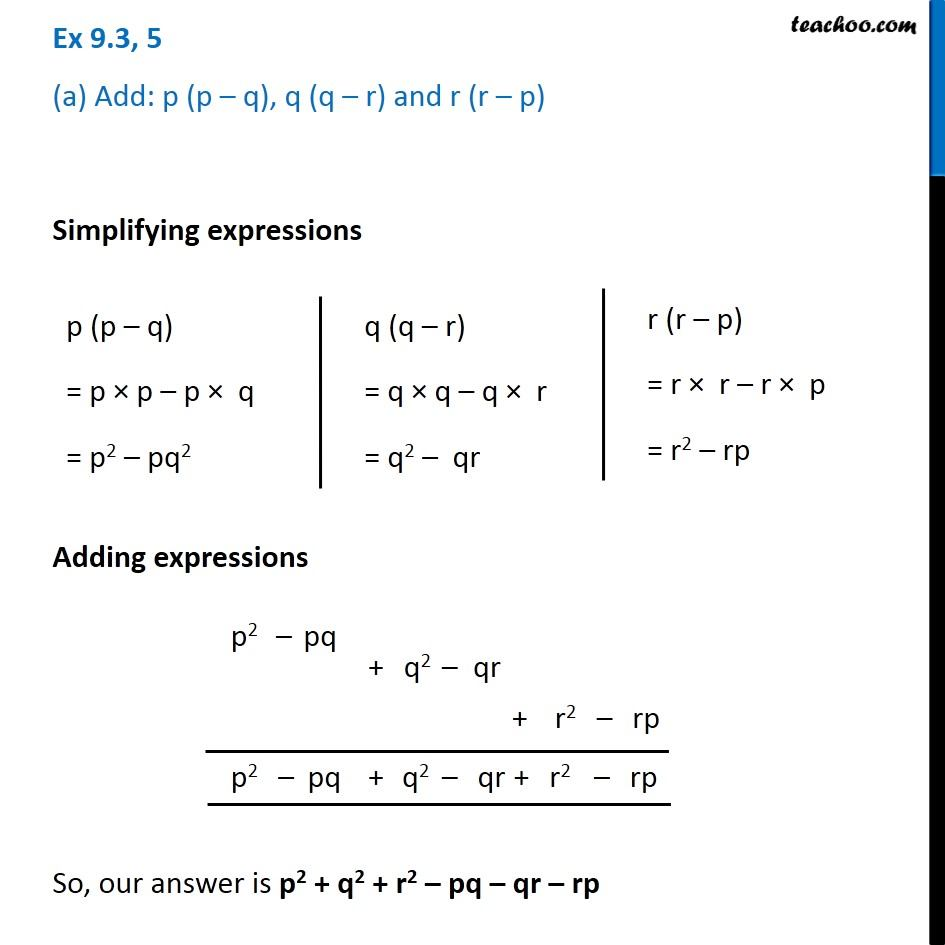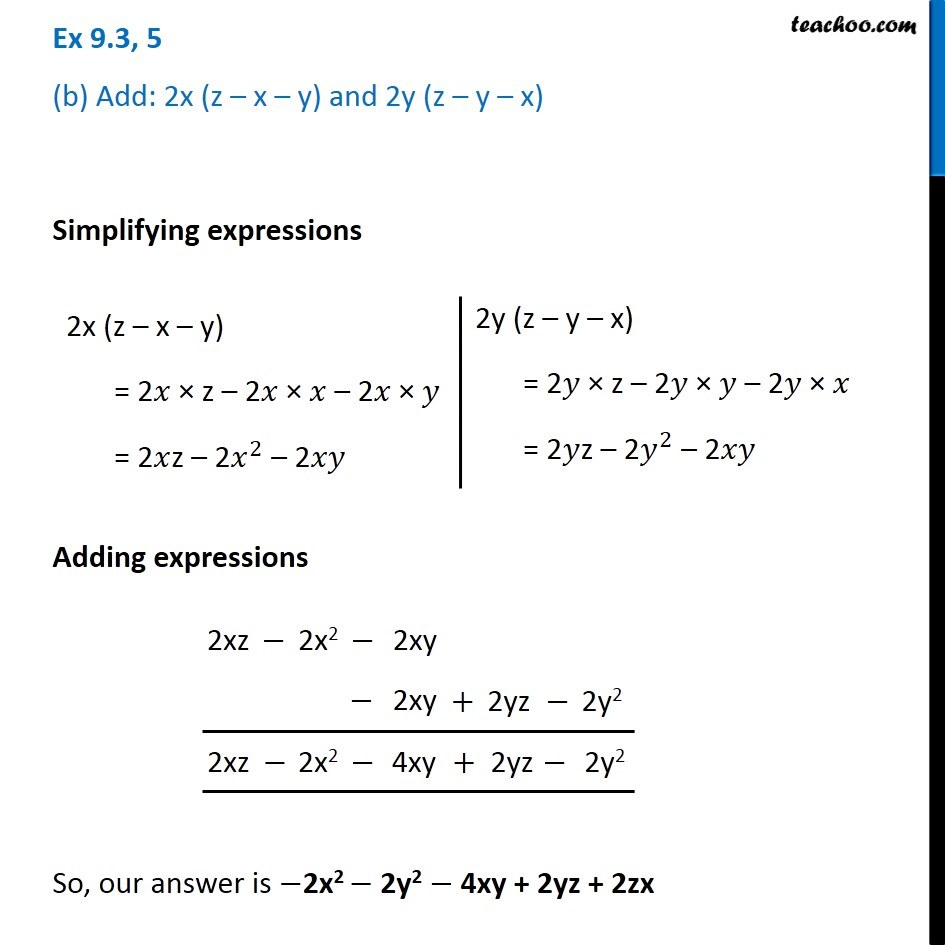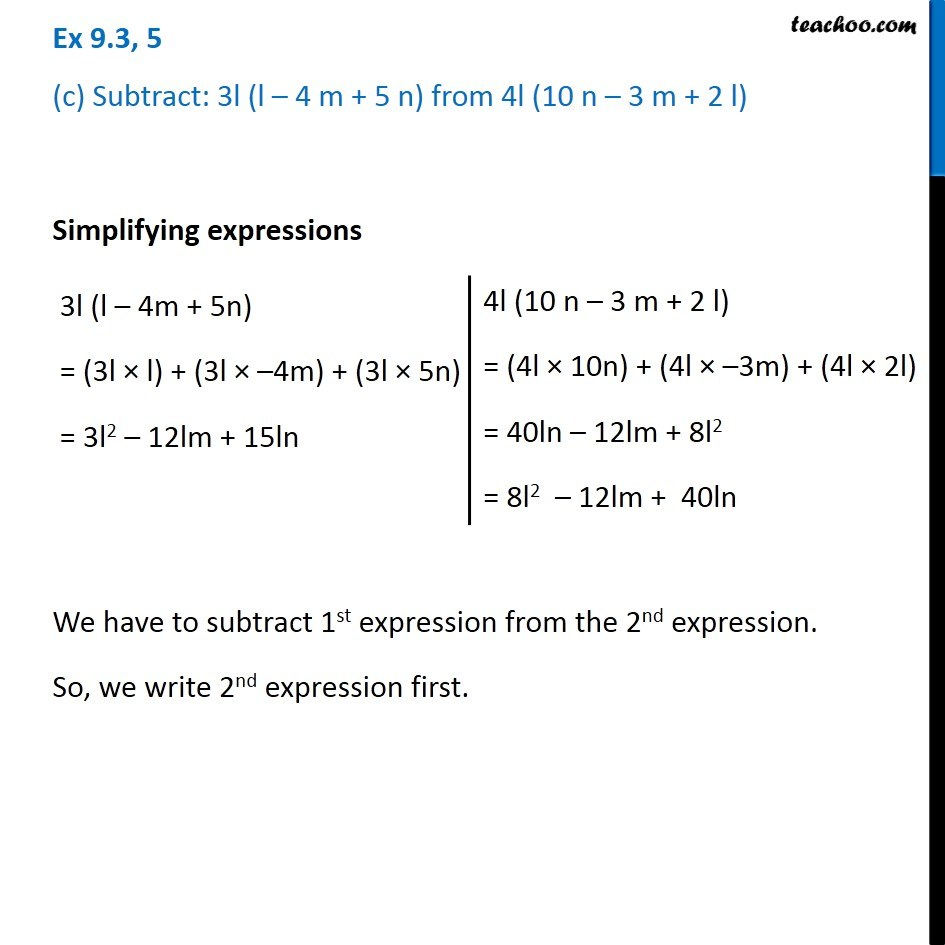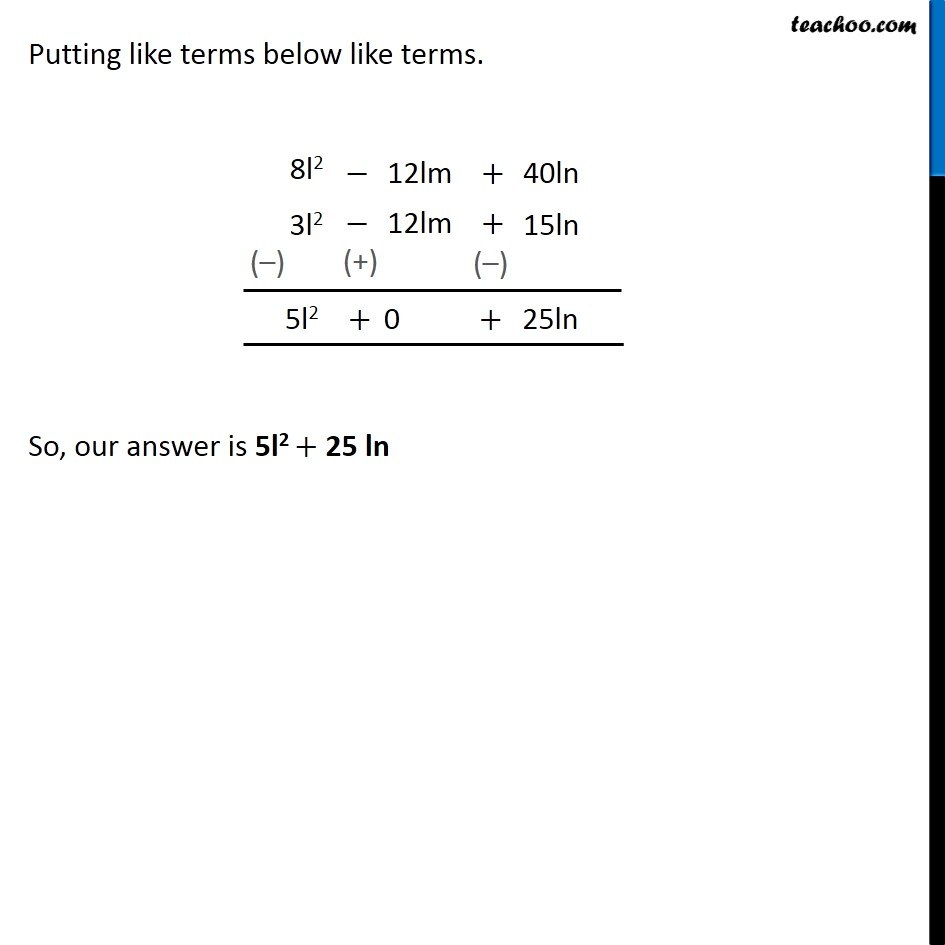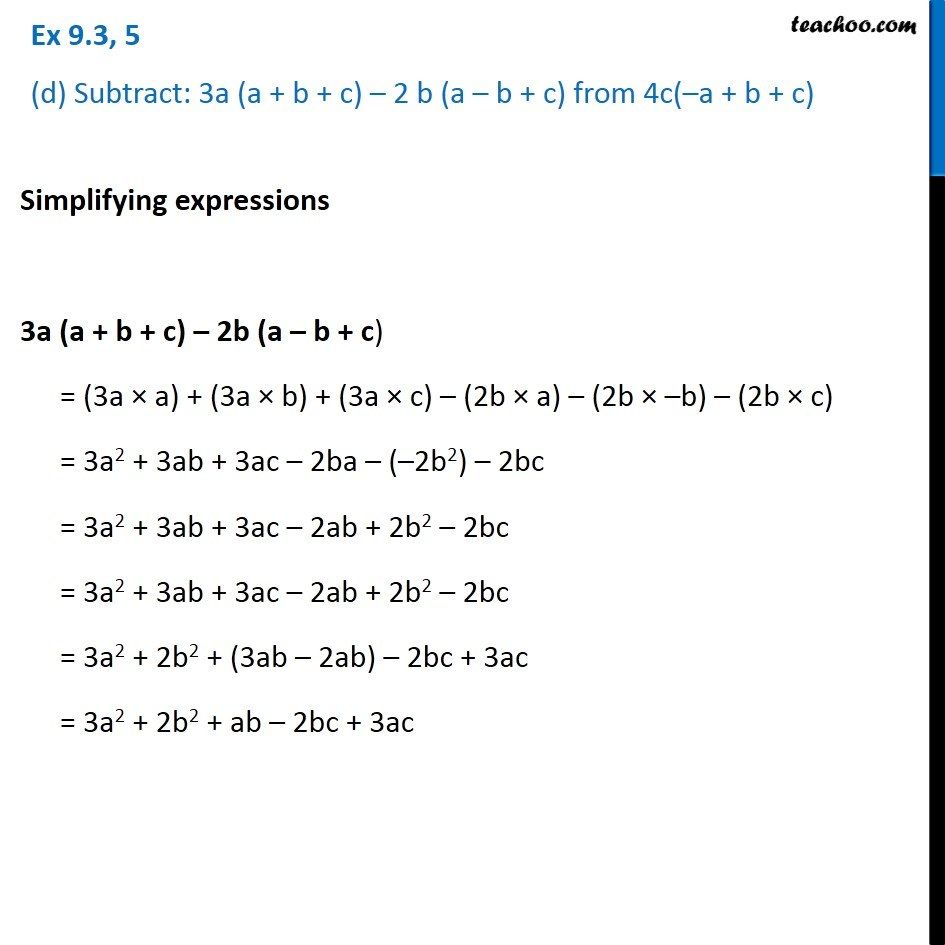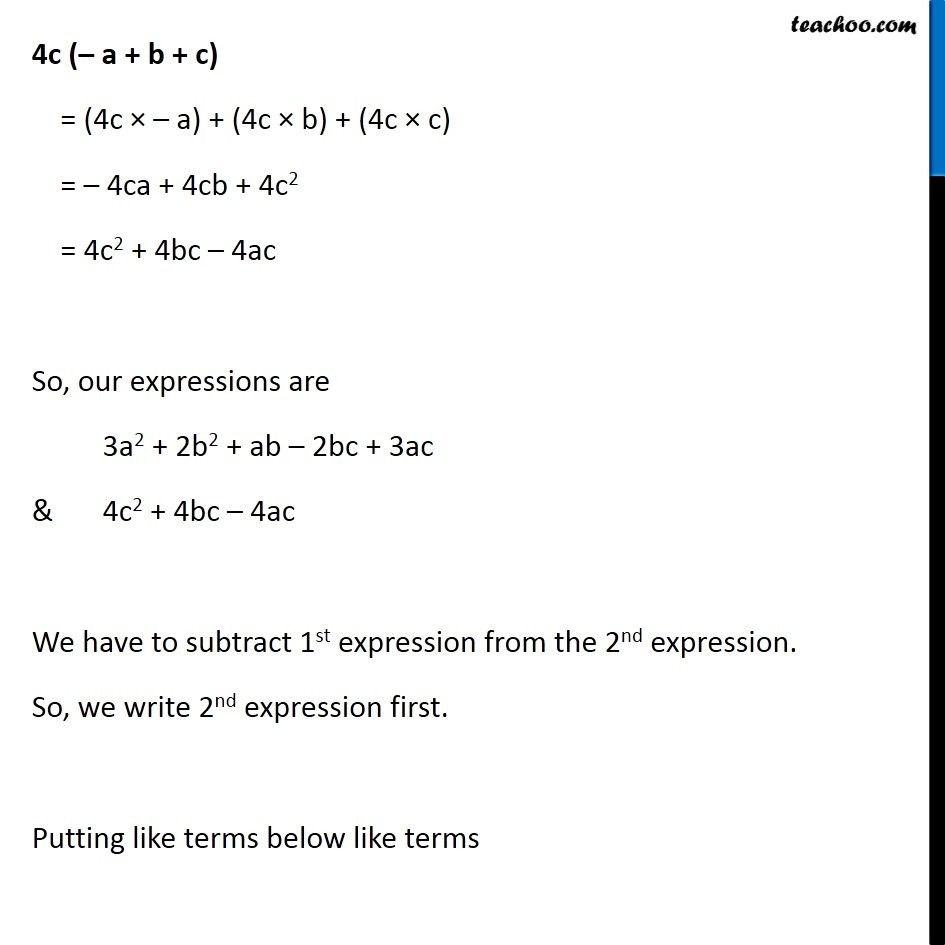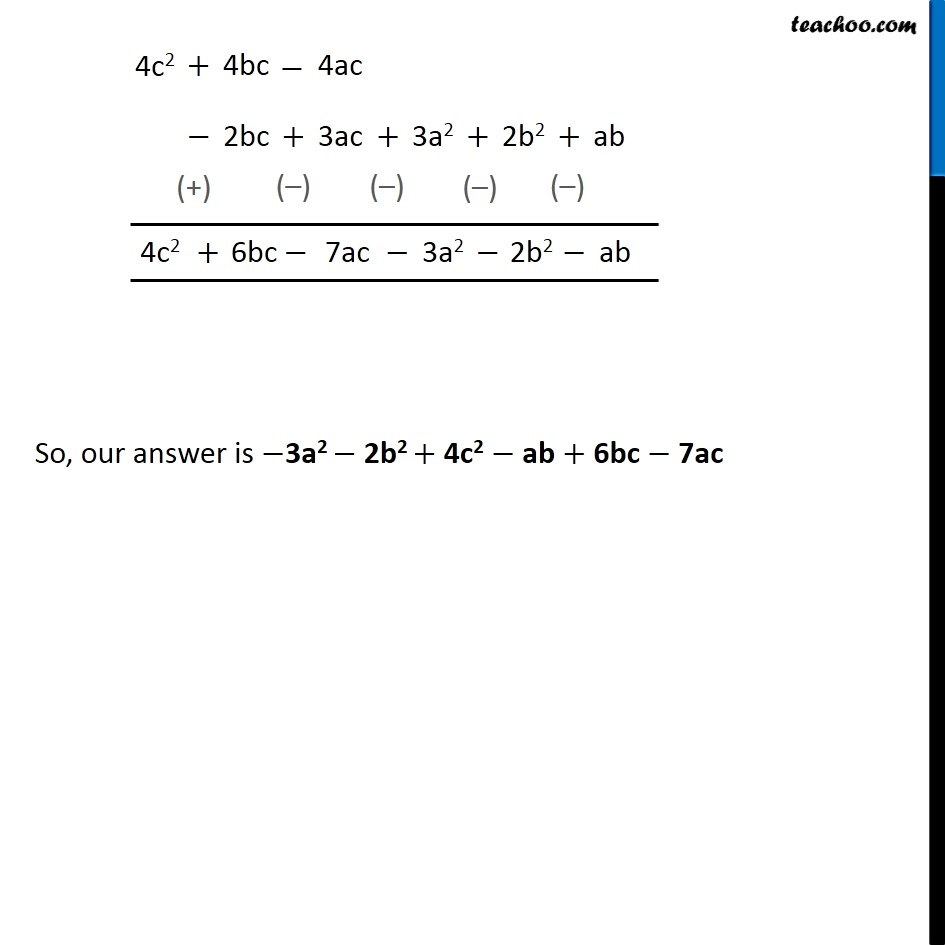Subscribe to our Youtube Channel - https://you.tube/teachoo

1. Chapter 9 Class 8 Algebraic Expressions and Identities
2. Serial order wise
3. Ex 9.3

Transcript

Ex 9.3, 5 (a) Add: p (p – q), q (q – r) and r (r – p) Simplifying expressions p (p – q) = p × p – p × q = p2 – pq2 q (q – r) = q × q – q × r = q2 – qr r (r – p) = r × r – r × p = r2 – rp So, our answer is p2 + q2 + r2 – pq – qr – rp Ex 9.3, 5 (b) Add: 2x (z – x – y) and 2y (z – y – x) Simplifying expressions 2x (z – x – y) = 2𝑥 × z – 2𝑥 × 𝑥 – 2𝑥 × 𝑦 = 2𝑥z – 2𝑥^2 – 2𝑥𝑦 2y (z – y – x) = 2𝑦 × z – 2𝑦 × 𝑦 – 2𝑦 × 𝑥 = 2𝑦z – 2𝑦^2 – 2𝑥𝑦 Adding expressions So, our answer is −2x2 − 2y2 − 4xy + 2yz + 2zx Ex 9.3, 5 (c) Subtract: 3l (l – 4 m + 5 n) from 4l (10 n – 3 m + 2 l) Simplifying expressions 3l (l – 4m + 5n) = (3l × l) + (3l × –4m) + (3l × 5n) = 3l2 – 12lm + 15ln 4l (10 n – 3 m + 2 l) = (4l × 10n) + (4l × –3m) + (4l × 2l) = 40ln – 12lm + 8l2 = 8l2 – 12lm + 40ln 4l (10 n – 3 m + 2 l) = (4l × 10n) + (4l × –3m) + (4l × 2l) = 40ln – 12lm + 8l2 = 8l2 – 12lm + 40ln Putting like terms below like terms. So, our answer is 5l2 + 25 ln Ex 9.3, 5 (d) Subtract: 3a (a + b + c) – 2 b (a – b + c) from 4c(–a + b + c)Simplifying expressions 3a (a + b + c) – 2b (a – b + c) = (3a × a) + (3a × b) + (3a × c) – (2b × a) – (2b × –b) – (2b × c) = 3a2 + 3ab + 3ac – 2ba – (–2b2) – 2bc = 3a2 + 3ab + 3ac – 2ab + 2b2 – 2bc = 3a2 + 3ab + 3ac – 2ab + 2b2 – 2bc = 3a2 + 2b2 + (3ab – 2ab) – 2bc + 3ac = 3a2 + 2b2 + ab – 2bc + 3ac 4c (– a + b + c) = (4c × – a) + (4c × b) + (4c × c) = – 4ca + 4cb + 4c2 = 4c2 + 4bc – 4ac So, our expressions are 3a2 + 2b2 + ab – 2bc + 3ac & 4c2 + 4bc – 4ac We have to subtract 1st expression from the 2nd expression. So, we write 2nd expression first. Putting like terms below like terms So, our answer is −3a2 − 2b2 + 4c2 − ab + 6bc − 7ac

Ex 9.3